www.batmath.it

### Final exercises

1. If t is a real number such that 0<t<2, calculate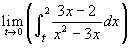.
2. Given the function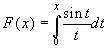, find the points where it gets a relative maximum. (The integral function is supposed to be extended so that it is everywhere continuous).
3. Solve the following inequality: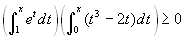.
4. f is a function continuous together with its first and second derivative; the tangents to the graph of f in the numbers a and b create an angle whose measure is, respectively, of π/3 and π/4 with he positive x-axis. Calculate the following two integrals: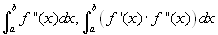.
5. Given the function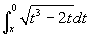, find the intervals where the function is increasing and those where the function is convex.
6. Given the function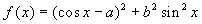, find the values of the numbers a and b such that f(0)=0 and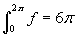. Graph the function.
7. Calculate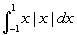.
8. Given the function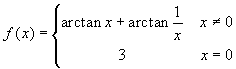, calculate its integral function with starting point -1. What can say about the derivative of this integral function?
9. By direct calculation of the integral find the derivative of the function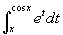. Find again the same derivative without calculating the integral.
10. Find the domain of the following functions:
•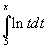.
•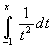.
•.
•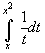.
•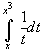.
11. Prove that if f is an integrable function over [-a,a] and f(-x)=-f(x) than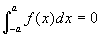. Give a geometrical interpretation of this result.
12. The integral of a function is unchanged if we alter the values of a fucntion in a finite number of points. However this is no more true if we alter the function in a countably infinite number of points. Proof this with an example (sugg.: use Dirichlet's function).
first published on january 07 2003 - last updated on september 01 2003